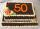# 144 steps

To get around the garden we have to take 144 steps. 8 steps less in width than in length. One step is 5 decimetres long. What are the dimensions of the garden? What is its perimeter and its area?

a =  20 m
b =  16 m
o =  72 m
S =  320 m2

### Step-by-step explanation:Did you find an error or inaccuracy? Feel free to write us. Thank you!Tips to related online calculators
Do you have a system of equations and looking for calculator system of linear equations?

#### You need to know the following knowledge to solve this word math problem:

We encourage you to watch this tutorial video on this math problem:

## Related math problems and questions:

• RectangleArea of rectangle is 3002. Its length is 41 larger than the width. What are the dimensions of the rectangle?
• Rectangular gardenThe perimeter of Peter's rectangular garden is 98 meters. The width of the garden is 60% shorter than its length. Find the dimensions of the rectangular garden in meters. Find the garden area in square meters.
• The rectangleDetermine the area of the rectangle where the length and width are in the ratio 5:2 and its length is 7.5 cm longer than its width. Determine also its length and its width.
• Dimensions of rectangleFind the dimensions of a rectangle given that its perimeter is 110 cm and its length is 1 cm more than twice its width.
• Dimensions 3A perimeter of a rectangular field is 96 meters. The length is four meters less than three times the width. Find the length and width (its dimensions).
• RectangleThe length of the rectangle is 12 cm greater than 3 times its width. What dimensions and area this rectangle has if ts circumference is 104 cm.
• The circumferenceThe circumference and width of the rectangle are in a ratio of 5: 1. its area is 216cm2. What is its length?
• Squares above sidesIn a right triangle, the areas of the squares above its sides are 169; 25 and 144. The length of its longer leg is:
• RectangleThe width of the rectangle is 65% of its length. Perimeter of the rectangle is 132 cm. Determine the dimensions of the rectangle.
• RectanglePerimeter of rectangle is 48 cm. Calculate its dimensions if they are in the ratio 5:3 (width:height)
• Perimeter of a rectangleIf the perimeter of a rectangle is 114 meters and the length is twice the width plus 6 meters, what are the length and width?
• Wire fenceThe wire fence around the garden is 160 m long. One side of the garden is three times longer than the other. How many meters do the individual sides of the garden measure?
• RectangleFind the dimensions of the rectangle, whose perimeter is 108 cm and the length is 25% larger than the width.
• PropertyThe length of the rectangle-shaped property is 8 meters less than three times of the width. If we increase the width 5% of a length and lendth reduce by 14% of the width it will increase the property perimeter by 13 meters. How much will the property cost
• Area of gardenIf the width of the rectangular garden is decreased by 2 meters and its length is increased by 5 meters, the area of the rectangle will be 0.2 ares larger. If the width and the length of the garden will increase by 3 meters, its original size will increas
• PlotThe length of the rectangle is 8, smaller than three times the width. If we increase the width by 5% of the length and the length is reduced by 14% of the width, the circumference of the rectangle will be increased by 30 m. What are the dimensions of the
• Two rectanglesI cut out two rectangles with 54 cm², 90 cm². Their sides are expressed in whole centimeters. If I put these rectangles together I get a rectangle with an area of 144 cm2. What dimensions can this large rectangle have? Write all options. Explain your calc